# Basic Tutorial Lesson 3: Analyzing a Basic Bipolar Junction Transistor Circuit

 Tutorial Project: Analyzing a Basic Bipolar Junction Transistor Circuit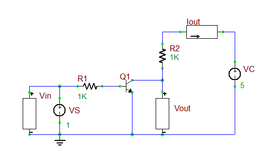Objective: In this project, you will build and analyze a simple RTL (Resistor-Transistor-Logic) inverter circuit using a bipolar junction transistor and will operate it as an amplifier. Concepts/Features: Bipolar Junction Transistor Voltmeter Ammeter DC Sweep Test Transient Test Small-Signal AC Analysis Minimum Version Required: All versions

## What You Will Learn

In this tutorial you will learn how to define, use and customize active devices such as bipolar junction transistors (BJT). Specifically, you will build a simple RTL (Resistor-Transistor-Logic) inverter circuit and perform DC, transient and AC analyses of your inverter circuit. You will also learn how to place and use voltmeters and ammeters. A picture of the final circuit is shown below:

## Choosing Parts and Drawing the Circuit

The following is a list of parts needed for this project:

Part Name Part Type Part Value
VS Voltage Source 1V
VC Voltage Source 5V
R1 Resistor 1k
R2 Resistor 1k
Q1 Generic NPN (BJT) Transistor beta = 150
Vin Vertical Voltmeter N/A
Vout Vertical Voltmeter N/A
Iout Horizontal Ammeter N/A

Choose the above parts either from Parts Menu or from the Parts Bin of the "Add Part" tab of the Toolbox. You can also use the convenient keyboard shortcuts for part placement. The keyboard shortcut for Generic NPN BJT is Q. The keyboard shortcuts for Voltmeter and Ammeter are Y and Alt+Y, respectively.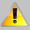Remember that once you choose a part and its ghost appears on the circuit, you can use the right mouse button to rotate it before placing the part at the final location.

Open the property dialog of each part to change its parameter values if necessary. The property dialog of the BJT transistor is shown in the opposite figure. Change the value of the "Forward Beta" parameter to 150. To do so, select the parameter from the table by highlighting it, enter the new value in the "Value" box and click the Accept Value button. Then, use the Wire Tool to connect all the parts as shown in the figure at the beginning of the tutorial. Don't forget to place a ground for your circuit.

## Running a DC Sweep Test

First, you will analyze the DC response of your RTL circuit to verify whether it behaves as an inverter. Bring up the Tests Toolbox, select the "DC Sweep" option and open the Test Setup Dialog. Enter the following sweep parameters:

 Source VS DC 0 5V 50m v(vout)Whenever you define a meter (Voltmeter, Ammeter or Wattmeter) in your circuit, it is automatically added to the Virtual Instrument Panel. The measured signal of the meter is also automatically added to the plot signal list.

Make sure that the Single Test tab is selected and the check box labeled "Graph" is checked in the "Output Results Options" section of the dialog. Before running the test, check the observables. For this lesson you need the output voltage only. Click the button labeled "Preset Graph Plots..." to open the Edit Plots List Dialog as shown in the figure below. Remove the input voltage and output current from the graph list using the button labeled <--Remove and leave the output voltage only.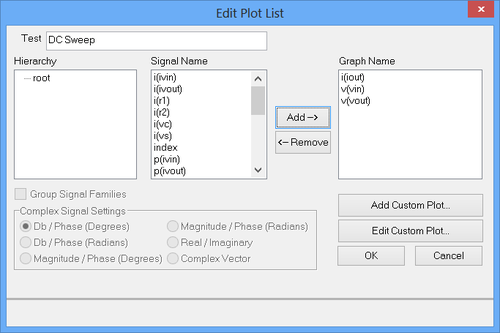Removing unwanted plots from the Edit Plot List.

Click the Run button. A graph of the output voltage vs. input voltage appears at the bottom of the Workshop as shown below. You will notice that the circuit does indeed invert the input. When the input is 0V, the output is 5V, and when the input is 5V, the output is 0V. Also, notice the steep slope of the graph when the input is around 0.8 Volts. This linear region displays the amplification potential of the bipolar junction transistor, which you will explore next.The variation of the output voltage of the RTL inverter vs. the input voltage

## Running a Transient Test

Next, you will perform a transient analysis of your RTL inverter circuit. Observing the output's response to a constant input is all but boring. So modify the voltage source VS to have sinusoidal transient properties rather than a constant DC. Double click on the voltage source to open its property dialog as shown in the figure below. Change the source's "Transient" property to "Sinusoidal". Set the "Offset Voltage" of the sinusoidal waveform to 0.80 Volts to bias the transistor in its amplifying region. Set the "Amplitude" to 0.01V and "Frequency" to 1kHz. As you can see from the figure, the input signal swings between 0.79V and 0.81V.

Bring up the Transient Test from the Tests Toolbox, and set the parameters for the test as follows:

 Start Time 0 2m 1u 1u v(vin), v(vout)

Don't forget to deselect the DC Sweep Test from the previous step of this tutorial lesson. In the "Output Results Options" section of the transient test setup dialog, click the button labeled "Preset Graph Plots..." to open the Edit Plots List Dialog. Make sure you have both the input voltage and output voltage in the graph table. Click the Run button to run the transient test. The following graph will appear at the bottom of the Workshop.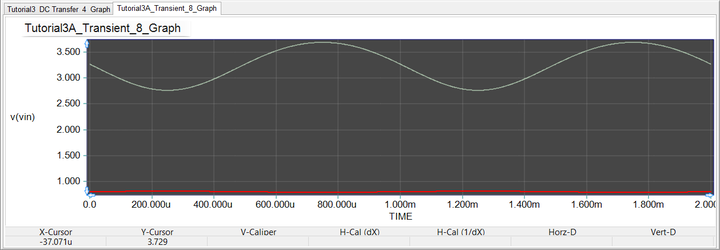The variation of the output voltage of the RTL inverter vs. the input voltage.

In the above graph, the red and silver-green plots represent the input and output voltages, respectively. Notice that there is no apparent distortion in the output signal. Since the graph is automatically zoomed to display all signals, the fluctuations of the input signal are barely visible. To better see the input voltage fluctuations, you can zoom to a selected plot. With the graph view active, open the "Edit Plots" tab of the Toolbox as shown below. At the top of the settings dialog, the two signals v(vin) and v(vout) are listed. There are also two checked boxes preceding their names, meaning that both plots are visible in the graph. Click on the input voltage signal to highlight it in the list table. Now the v(vin) signal is selected in the graph and its curve becomes a little thicker (highlighted on the graph). Then, click the "Zoom to Selected Plot"button of the Graph Toolbar. The graph will be automatically zoomed to spotlight the selected plot as shown below. However, the output signal goes out of sight. Note that "Zoom to Selected Plot" is a mode that persists until you deselect the corresponding button of the Graph Toolbar.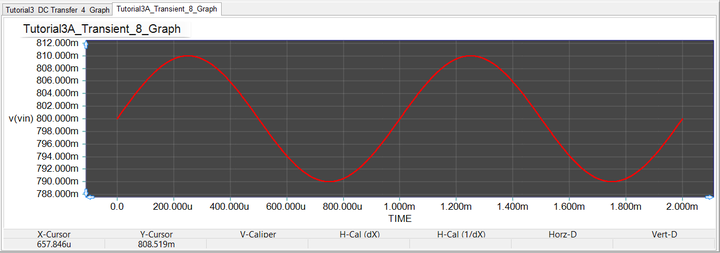The variation of the output voltage of the RTL inverter vs. the input voltage.

In order to get the graph back to its original state where you could see all plots, click the "Scale to Fit All Plots"button of the Graph Toolbar. You will use this button quite often.

You already know that the (AC) amplitude of the input voltage is 0.01V swinging about a DC bias of 0.8V. In order to better examine the output voltage and estimate the amplification gain of your inverter circuit, next you zoom the graph to the second plot v(vout). Follow the same procedure to highlight the output voltage signal in Edit Plots tab of the Toolbox and "Zoom to Selected Plot" in the Graph Toolbar. You can easily read the peak-to-peak swing of the output voltage using RF.Spice's useful "Delta Line Tool". This tool can measure the X and Y difference between any two points on any graph. While your graph has been zoomed to the output voltage signal, click the "Delta Line Mode"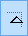button of the Graph Toolbar. Place the mouse at the negative peak of the graph and click the left mouse button and then drag it to its next positive peak. The Delta X and Delta Y values are displayed in the Status Bar. The peak-to-peak amplitude is read as 0.934V, corresponding to a peak amplitude of 0.467V. To summarize the results of the analysis, this circuit amplifies a small signal input 46.7 times when the input's offset voltage is biased around 0.8 volts.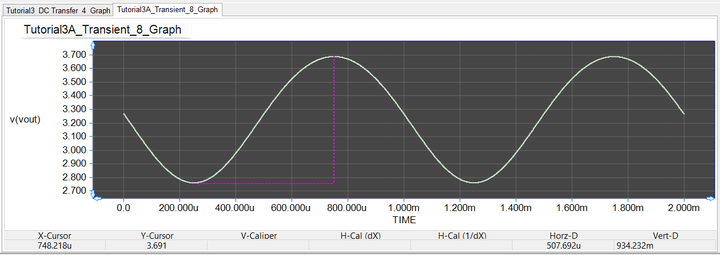The variation of the output voltage of the RTL inverter vs. the input voltage.

## Running an AC Sweep Test

Running a transient analysis with sinusoidal waveforms is not very informative except for the fact that you will see the temporal period variation of signals over time. For harmonic waveforms, AC sweep is usually the choice test. From the previous lesson, remember that you have to designate your AC source explicitly. In this lesson, the input voltage source VS will serve as the AC source. Double-click on the voltage source to open its property dialog. Open the second tab of the dialog labeled "Small-Signal AC & Distortion". At the top of the dialog, enter a DC voltage of 0.8V for the "Small-Signal Offset". Keep in mind that your BJT transistor will not amplify if not properly biased. Check the "Use" box of the AC source and set its AC properties to an "Amplitude" of 0.01V and a "Phase" of 0 degrees. Close the source dialog.

Next, bring up the AC Sweep Test from the Tests Toolbox, and use one of the preset frequency ranges by clicking the button labeled "1Hz-1MHz". Don't forget to deselect the Transient Test from the previous step of this tutorial lesson. In the "Output Results Options" section of the AC sweep test setup dialog, click the button labeled "Preset Graph Plots..." to open the Edit Plots List Dialog. Make sure you have both the input voltage VDB(vin) and output voltage VDB(vout) in the graph table.

 Start Frequency 1 1Meg 20 Decade VDB(vin), VDB(vout)In AC Sweep Test, the default signal plots are expressed in dB-magnitude and phase in degrees.

Click the Run button to run the AC sweep test. The bode plots of the input and output voltages appear at the bottom of the Workshop. To better use the graph, you will make some changes to it. First, open the "Edit Plots" tab of the Toolbox as shown below. Select the output signal and change its color to yellow. Pick the color from the drop-down list labeled "Plot Color". Then, switch to the "Edit Axes" tab of the Toolbox as shown below. Select "Left Axis" from the top of the settings dialog. In the "Scale" tab of the dialog, uncheck the "Auto" check box and enter values -40 and 0 for the "Min" and "Max" values, respectively. make sure you press the button labeled "Apply Above Changes". This will re-scale the graph between -40dB and 0dB as shown in the figure below.

The input signal has an AC amplitude of 10mV or 0.01V. In the dB scale, this is equivalent to 20*log10(0.01) = -40dBV. The output voltage of your circuit is measured to be -6.683dBV. This yield a gain of G(dB) = -6.683dBV - (-40dBV) = 33.317dB. To scale this number back to the linear scale, you will get a voltage gain of GV = 10^(33.317/20) = 46.33, which is very close to your estimate from the previous section of this tutorial lesson.